Achievable Feedback Performance

Signals, Systems and Control Department
Faculty of Mathematical Sciences
University of Twente
The Netherlands

Introduction
Many of the properties of the MIMO feedback loop of Fig. 1 are characterized by its sensitivity matrix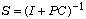and complementary sensitivity matrix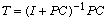These properties include the sensitivity of the feedback loop to disturbances and measurement noise, its response to reference inputs, and its stability and performance robustness (Kwakernaak, 1995).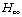optimization is a powerful tool for shaping the sensitivity functions so that all the design requirements are satisfied. In particular the mixed sensitivity problem is a useful design paradigm. The Polynomial Toolbox contains the routine mixeds for solving SISO mixed sensitivity problems.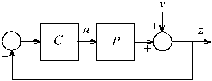Fig. 1. LTI feedback system

Especially for complex design problems it is highly recommended to devote some time to exploratory analysis before attempting the actual design. This exploratory analysis serves to reveal the inherent possibilities and limitations of the control system. Part of this exploratory analysis always is the computation of the poles and zeros of the plant. Any right-half plane zeros and poles that are present impose essential constraints on the closed-loop bandwidth that may be achieved or is necessary (again see Kwakernaak, 1995, for a review).

For MIMO systems the open-loop pole and zero locations do not fully reveal the design limitations. For instance, in the SISO case the presence of a nearly cancelling pole-zero pair in the right-half plane predicts poor performance, with high peaks in the sensitivity functions. The closer the pole and zero are, the higher the peak. In the case of MIMO systems the pole of such a pair may occur in a different ”channel” than the zero so that the pole and zero do not interact and their adverse effects are not amplified such as in the SISO case.

To reveal the a priori design limitations more fully it may be useful to compute the minimal peak values of the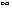-norms of the sensitivity functions S and T. This is the purpose of this demo. Polynomial techniques lend themselves very well for the computation of these bounds and explicitly reveal their relation to the open-loop zero and pole locations. The peak values themselves are lower bounds for the peak values that are obtained for more realistic designs matched to the design specifications.

SISO minimal peak values for the sensitivities
For the SISO case the minimal peak values are derived in Kwakernaak (1985). We assume that the plant has the transfer function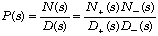The polynomial N is the plant numerator polynomial and the polynomial D is the plant denominator polynomial.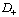and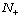are polynomials whose roots have strictly positive part and hence lie in the open right-half complex plane. The roots of the polynomials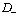and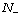all have nonpositive real part.

The minimal peak value of the-norm of the sensitivity function S in the SISO case equals the minimal peak value of the magnitude of the sensitivity function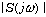,. This minimal norm may be found by solving a specialoptimization problem, namely that of minimizing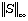. The solution of this minimum sensitivity problem follows by solving the polynomial equation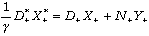for the scalarand the polynomials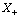and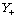. Ifhas degree d andhas degree n thenhas degree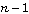andhas degree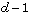. As we shall see this equation is equivalent to a generalized eigenvalue problem. The compensator that solves the minimum sensitivity problem has the transfer function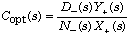If the plant is strictly proper then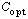is nonproper. The optimal sensitivity function is given by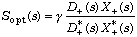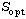has the so-called equalizing property, that is,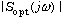is constant for all real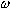and equals. The minimal peak value hence is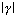.

The properties of the minimum peak value may be summarized as follows:

• If the plant has no right-half plane zeros then, which is achieved by an infinite-gain compensator.
• If the plant has no right-half plane poles but at least one right-half plane zero then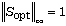, which is obtained by taking.
• If the plant has at least one right-half plane zero and at least one right-half plane pole then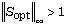.
• If the plant has a coinciding right-half plane pole-zero pair then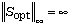.

For the minimal peak value of the complementary sensitivity function T similar results hold. The critical equation that needs to be solved now isand the optimal complementary function is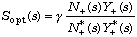This is the summary of the results for the minimization of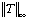:

• If the plant has no right-half plane poles then, which is achieved by an infinite-gain compensator.
• If the plant has no right-half plane zeros but at least one right-half plane pole then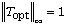, which is obtained by taking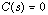.

• If the plant has at least one right-half plane zero and at least one right-half plane pole then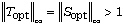.
• If the plant has a coinciding right-half plane pole-zero pair thenNote that minimal sensitivity and minimal complementary sensitivity are not simultaneously achieved by the same compensator.

SISO computation of the minimum peak value
The only situation where some serious computation needs to be done once the open-loop poles and zeros are available arises when the plant has both right half plane zeros and poles. We then need to solve the polynomial equationThe solution of this problem provides the minimal peak value of both the sensitivity and the complementary sensitivity function. Ifhas degree d andhas degree n thenhas degreeandhas degree. Writeandin terms of their coefficients as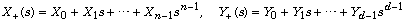and introduce the column vectors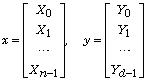Then it may be verified that the polynomial equation we need to solve is equivalent to the matrix equation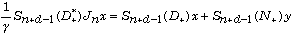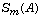denotes the (column) Sylvester resultant matrix of order m of the polynomial A, and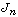is the matrixTo show that amounts to a generalized eigenvalue problem we rearrange it in the form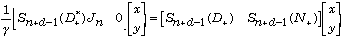This is equivalent to the equation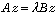whereWe need the solution of that corresponds to the real eigenvalue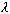with the smallest size. The minimal peak sensitivity equals the inverse of the magnitude of the smallest eigenvalue.

The macro minsens
We develop a new Polynomial Toolbox function minsens that computes the minimal peak value of the sensitivity functions. Its input arguments are the numerator polynomial N and the denominator polynomial D of the SISO plant.

As we develop the macro we test it for the plant with transfer function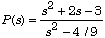We input the data accordingly as

The first few lines of the m-file are

% minsens
% The function
% p = minsens(N,D)
% computes the minimum peak value of the sensitivity and
% complementary sensitivity functions for the SISO plant
% with transfer function P = N/D that may be achieved by
% feedback
function p = minsens(N,D)

This provides the help text, and declares the function, its input arguments and its output arguments.

Normally a sequence of tests needs to follow this preamble to check whether N and D are really scalar polynomials but we dispense with this for the purpose of this demo.

Given the numerator and denominator polynomials we now compute their roots and use these to define the polynomialsand:

% Compute the polynomials Nplus and Dplus whose roots are
% the roots of N and D, respectively, with positive real parts
rootsN = roots(N); rootsNplus = rootsN(find(real(rootsN)>0));
Nplus = mat2pol(poly(rootsNplus));
rootsD = roots(D); rootsDplus = rootsD(find(real(rootsD)>0));
Dplus = mat2pol(poly(rootsDplus));
keyboard

The Matlab command poly is used to construct the polynomials Nplus and Dplus from their roots after which they are converted to Polynomial Toolbox format with the Toolbox command mat2pol. While developing the macro we end it with the keyboard command so that the results at that point may be inspected. In the present case typing the command

minsens(N,D)

results in the output

Editing this to

K» Nplus, Dplus

and ending the line with a return results in the output

Nplus =
-1 + s

Dplus =
-0.67 + s

We now include two tests to see whether the peak value is either 0 or 1.

% Check whether p = 0 or p = 1
if isempty(Nplus)
p = 0; return
elseif isempty(Dplus)
p = 1; return
end

For the example that we are pursuing both tests fail so we are in the situation where the generalized eigenvalue problem needs to be solved. We first set it up.

% Solve the generalized eigenvalue problem
A = [ sylv(Dplus,'col',n-1) sylv(Nplus,'col',d-1) ];
J = 1;
for i = 2:n
J(i,i) = -1*J(i-1,i-1);
end
B = [ sylv(Dplus','col',n-1)*J zeros(n+d,d) ];

Only one more line is needed to complete the macro:

p = 1/min(abs(eig(A,B)));

Calling

minsens(N,D)

results for the example at hand in

ans =

5.0000

We test a few more examples

• No right-half plane zeros:
• One right-half plane zero but no right-half plane poles:

minsens(s-1,s+1)
ans =
1

• Coinciding right-half plane pole-zero pair:

minsens(s-1,s-1)
Warning: Divide by zero.
ans =
Inf

MIMO minimal peak values for the sensitivities
For the MIMO case no simple results to compute the minimal peak sensitivity such as for the SISO case are available. The only option seems to be to compute a full solution of the minimum sensitivity problem. The Polynomial Toolbox provides the routine dssrch for this purpose. It solves the standardoptimization problem, is numerically well-conditioned, fast, computes optimal solutions accurately and reliably, and can handle nonproper generalized plants as well as problems where the optimal compensator is nonproper. The routine requires the generalized plant of the standard problem to be in descriptor form. The Toolbox supplies the necessary conversion routines from polynomial matrix fraction form to descriptor form and vice-versa.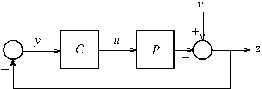Fig. 2. Minimum sensitivity problem

To bring the minimum sensitivity problem into standard form we consider the block diagram of Fig. 2. When the loop is opened the signals are related as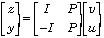This defines the generalized plant of the standard problem as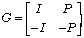If the plant P has the left coprime representationthen the generalized plant has the left coprime representation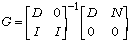After converting this left coprime fraction to descriptor form the routine dssrch may be called to solve theoptimization problem.

MIMO example
We generate a random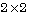plant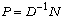by the commands

D = prand([1;2],2,2,'int')
D =
-8 + s 1 - 6s
6 + 6s 2 + s - s^2

N = prand([1;2],2,2,'int')
N =
-3 + 11s -1 + s
5 -4 + s - 7s^2

The zeros and poles of the plant are

Zeros = roots(N)
Zeros =
0.0379 + 0.8051i
0.0379 - 0.8051i
0.3399

Inspection shows that the plant has both right-half plane poles and zeros so by analogy to the SISO problem we expect a minimum peak sensitivity of at least 1.

We first convert the generalized plant into descriptor form:

Dg = [D zeros(2,2); eye(2,2) eye(2,2)];
Ng = [D N; zeros(2,2) zeros(2,2)];
[A,B,C,D,E] = lmf2dss(Ng,Dg)

A =
8 -47 0
-6 37 1
-6 38 0

B =
0 0 85.0000 336.0000
0 0 -66.0000 -264.0000
0 0 -61.0000 -276.0000

C =
1 -6 0
0 -1 0
-1 6 0
0 1 0

D =
1.0000 0 11.0000 43.0000
0 1.0000 0 7.0000
-1.0000 0 -11.0000 -43.0000
0 -1.0000 0 -7.0000

E =
1 0 0
0 1 0
0 0 1

The solution of theproblem is initiated by typing

[Ak,Bk,Ck,Dk,Ek,gopt,clpoles] = dssrch(A,B,C,D,E,2,2,0.5,5)

The resulting output is

Ak =
0.5209 1.0787
-0.9706 -0.1370

Bk =
-0.9241 -9.2055
1.7746 -0.6237

Ck =
0.0410 -0.0035
-0.1003 0.0981

Dk =
-0.3750 0.0976
0.0547 -0.0142

Ek =
1 0
0 1

gopt =
1.1595

clpoles =
-45.5167 + 0.0000i
-0.1755 - 0.9418i
-0.1755 + 0.9418i
-0.4833 - 0.0000i
-1.0000

We observe that the minimal norm is 1.1595. The fact that this number is not all that much greater than 1 indicates that a design without exaggerated peaking of the sensitivity functions is possible as long as the design specifications — in particular the desired bandwidth — are compatible with the limitations imposed by the right-half plane zeros and poles of the plant.